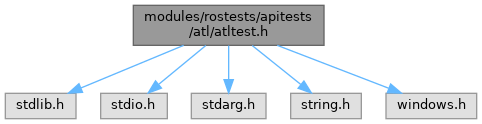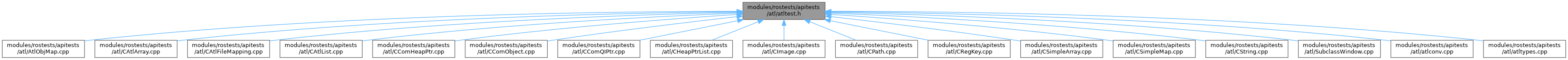ReactOS 0.4.15-dev-6054-gbddd8b0
atltest.h File Reference
`#include <stdlib.h>`
`#include <stdio.h>`
`#include <stdarg.h>`
`#include <string.h>`
`#include <windows.h>`
Include dependency graph for atltest.h:This graph shows which files directly or indirectly include this file:Go to the source code of this file.

## Macros

#define ok(value, ...)

#define ok_(x1, x2)   atltest_set_location(x1,x2); atltest_ok

#define skip(...)

#define trace   printf

#define START_TEST(x)

#define ok_hex(expression, result)

#define ok_dec(expression, result)

#define ok_ptr(expression, result)

#define ok_size_t(expression, result)

#define ok_char(expression, result)   ok_hex(expression, result)

#define ok_err(error)    ok(GetLastError() == (error), "Wrong last error. Expected " #error ", got 0x%lx\n", GetLastError())

#define ok_str(x, y)    ok(strcmp(x, y) == 0, "Wrong string. Expected '%s', got '%s'\n", y, x)

#define ok_wstr(x, y)    ok(wcscmp(x, y) == 0, "Wrong string. Expected '%S', got '%S'\n", y, x)

#define ok_long(expression, result)   ok_hex(expression, result)

#define ok_int(expression, result)   ok_dec(expression, result)

#define ok_ntstatus(status, expected)   ok_hex(status, expected)

#define ok_hdl   ok_ptr

## Functions

void atltest_set_location (const char *file, int line)

void atltest_ok (int value, const char *fmt,...)

void atltest_skip (const char *fmt,...)

static void atltest_start_test (void)

int main (void)

charwine_dbgstr_w (const wchar_t *wstr)

## Variables

int g_atltest_executed = 0

int g_atltest_failed = 0

int g_atltest_skipped = 0

const charg_atltest_file = NULL

int g_atltest_line = 0

const charg_atltest_name

## ◆ ok

 #define ok ( value, ... )
Value:
do { \
atltest_set_location(__FILE__, __LINE__); \
atltest_ok(value, __VA_ARGS__); \
} while (0)
Definition: pdh_main.c:94

Definition at line 57 of file atltest.h.

## ◆ ok_

 #define ok_ ( x1, x2 ) atltest_set_location(x1,x2); atltest_ok

Definition at line 61 of file atltest.h.

## ◆ ok_char

 #define ok_char ( expression, result ) ok_hex(expression, result)

Definition at line 122 of file atltest.h.

## ◆ ok_dec

 #define ok_dec ( expression, result )
Value:
do { \
int _value = (expression); \
ok(_value == (result), "Wrong value for '%s', expected: " #result " (%d), got: %d\n", \
#expression, (int)(result), _value); \
} while (0)
GLuint64EXT * result
Definition: glext.h:11304

Definition at line 101 of file atltest.h.

## ◆ ok_err

 #define ok_err ( error ) ok(GetLastError() == (error), "Wrong last error. Expected " #error ", got 0x%lx\n", GetLastError())

Definition at line 124 of file atltest.h.

## ◆ ok_hdl

 #define ok_hdl   ok_ptr

Definition at line 136 of file atltest.h.

## ◆ ok_hex

 #define ok_hex ( expression, result )
Value:
do { \
int _value = (expression); \
ok(_value == (result), "Wrong value for '%s', expected: " #result " (0x%x), got: 0x%x\n", \
#expression, (int)(result), _value); \
} while (0)

Definition at line 94 of file atltest.h.

## ◆ ok_int

 #define ok_int ( expression, result ) ok_dec(expression, result)

Definition at line 134 of file atltest.h.

## ◆ ok_long

 #define ok_long ( expression, result ) ok_hex(expression, result)

Definition at line 133 of file atltest.h.

## ◆ ok_ntstatus

 #define ok_ntstatus ( status, expected ) ok_hex(status, expected)

Definition at line 135 of file atltest.h.

## ◆ ok_ptr

 #define ok_ptr ( expression, result )
Value:
do { \
void *_value = (expression); \
ok(_value == (result), "Wrong value for '%s', expected: " #result " (%p), got: %p\n", \
#expression, (void*)(result), _value); \
} while (0)

Definition at line 108 of file atltest.h.

## ◆ ok_size_t

 #define ok_size_t ( expression, result )
Value:
do { \
size_t _value = (expression); \
ok(_value == (result), "Wrong value for '%s', expected: " #result " (%Ix), got: %Ix\n", \
#expression, (size_t)(result), _value); \
} while (0)

Definition at line 115 of file atltest.h.

## ◆ ok_str

 #define ok_str ( x, y ) ok(strcmp(x, y) == 0, "Wrong string. Expected '%s', got '%s'\n", y, x)

Definition at line 127 of file atltest.h.

## ◆ ok_wstr

 #define ok_wstr ( x, y ) ok(wcscmp(x, y) == 0, "Wrong string. Expected '%S', got '%S'\n", y, x)

Definition at line 130 of file atltest.h.

## ◆ skip

 #define skip ( ... )
Value:
do { \
atltest_set_location(__FILE__, __LINE__); \
atltest_skip(__VA_ARGS__); \
} while (0)

Definition at line 64 of file atltest.h.

## ◆ START_TEST

 #define START_TEST ( x )
Value:
const char *g_atltest_name = #x; \
static void atltest_start_test(void)
static void atltest_start_test(void)
const char * g_atltest_name
GLint GLint GLint GLint GLint x
Definition: gl.h:1548

Definition at line 75 of file atltest.h.

## ◆ trace

 #define trace   printf

Definition at line 70 of file atltest.h.

## ◆ atltest_ok()

 void atltest_ok ( int value, const char * fmt, ... )

Definition at line 32 of file atltest.h.

33{
34 va_list va;
35 va_start(va, fmt);
36 if (!value)
37 {
39 vprintf(fmt, va);
41 }
43 va_end(va);
44}
char * va_list
Definition: acmsvcex.h:78
#define va_end(ap)
Definition: acmsvcex.h:90
#define va_start(ap, A)
Definition: acmsvcex.h:91
int g_atltest_executed
Definition: atltest.h:19
int g_atltest_failed
Definition: atltest.h:20
int g_atltest_line
Definition: atltest.h:24
const char * g_atltest_file
Definition: atltest.h:23
#define printf
Definition: freeldr.h:94
_Check_return_opt_ _CRTIMP int __cdecl vprintf(_In_z_ _Printf_format_string_ const char *_Format, va_list _ArgList)
Definition: dsound.c:943

## ◆ atltest_set_location()

 void atltest_set_location ( const char * file, int line )

Definition at line 26 of file atltest.h.

27{
30}
Definition: fci.c:127
Definition: parser.c:49

## ◆ atltest_skip()

 void atltest_skip ( const char * fmt, ... )

Definition at line 46 of file atltest.h.

47{
48 va_list va;
49 va_start(va, fmt);
50 printf("%s (%d): test skipped: ", g_atltest_file, g_atltest_line);
51 vprintf(fmt, va);
53 va_end(va);
54}
int g_atltest_skipped
Definition: atltest.h:21

## ◆ atltest_start_test()

 static void atltest_start_test ( void )
static

Referenced by main().

## ◆ main()

 int main ( void )

Definition at line 79 of file atltest.h.

80{
82 printf("%s: %d tests executed (0 marked as todo, %d failures), %d skipped.\n",
84 return g_atltest_failed;
85}

## ◆ wine_dbgstr_w()

 char * wine_dbgstr_w ( const wchar_t * wstr )

Definition at line 87 of file atltest.h.

88{
89 static char buf;
91 return buf;
92}
#define NULL
Definition: types.h:112
#define CP_ACP
Definition: compat.h:109
#define WideCharToMultiByte
Definition: compat.h:111
GLenum GLuint GLenum GLsizei const GLchar * buf
Definition: glext.h:7751
#define _countof(array)
Definition: sndvol32.h:68

## ◆ g_atltest_executed

 int g_atltest_executed = 0

Definition at line 19 of file atltest.h.

Referenced by atltest_ok(), and main().

## ◆ g_atltest_failed

 int g_atltest_failed = 0

Definition at line 20 of file atltest.h.

Referenced by atltest_ok(), and main().

## ◆ g_atltest_file

 const char* g_atltest_file = NULL

Definition at line 23 of file atltest.h.

Referenced by atltest_ok(), atltest_set_location(), and atltest_skip().

## ◆ g_atltest_line

 int g_atltest_line = 0

Definition at line 24 of file atltest.h.

Referenced by atltest_ok(), atltest_set_location(), and atltest_skip().

## ◆ g_atltest_name

 const char* g_atltest_name
extern

Referenced by main().

## ◆ g_atltest_skipped

 int g_atltest_skipped = 0

Definition at line 21 of file atltest.h.

Referenced by atltest_skip(), and main().